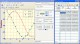Rt-Plot 2.8.10.83 Rt-Plot is a tool to generate Cartesian X/Y-plots from scientific data. SharewareRegression 2.1 Regression lets you perform complex regression analyses with extreme ease, making it perfect for classroom use or anywhere else non-statisticians might need to perform regressions. Shareware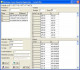CurveFitter 4.5.22 CurveFitter performs regression analysis to estimate values of parameters. Shareware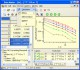DMFitter ActiveX control 11.8.0.386 Linear and nonlinear (Levenberg-Marquardt) curve fitting engine FreewareMultiple Regression Analysis and Forecasting 1.0 The Multiple Regression Analysis and Forecasting template provides a solid basis for identifying value drivers and forecasting time series data. Demo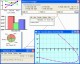UltimaCalc 4.1.941 Algebraic calculator. User functions. Charts. Regression. Std Deviation. Plots. Shareware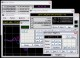Kalkulator 2.41 Advanced Sci/Eng calculator, from simple expressions to differential equations SharewareWebCab Probability and Stat for .NET 3.3 Statistics, Discrete Prob, Distributions, Hypo. testing, Correlation,Regression Demo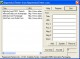Regression Tester 1.04 Website and Web Application Testing. SharewareWebCab Probability and Stat (J2SE Ed.) 3.3 Statistics, Discrete Prob, Distributions, Hypo. testing, Correlation,Regression Demo